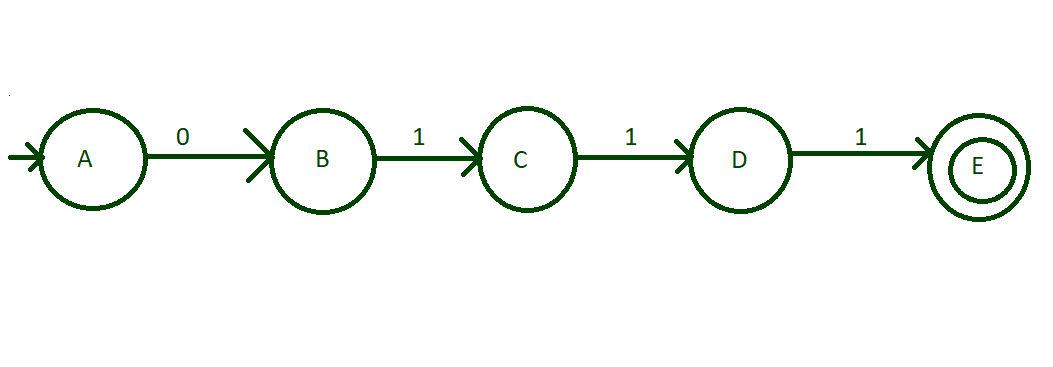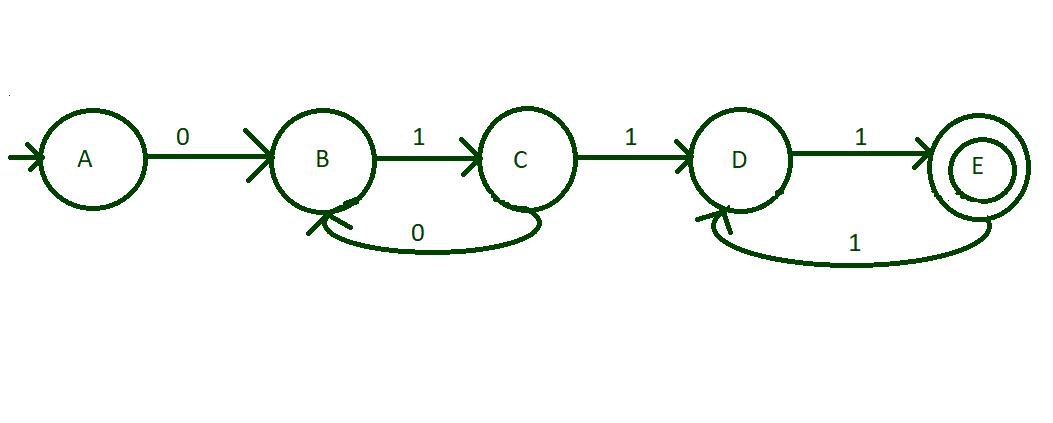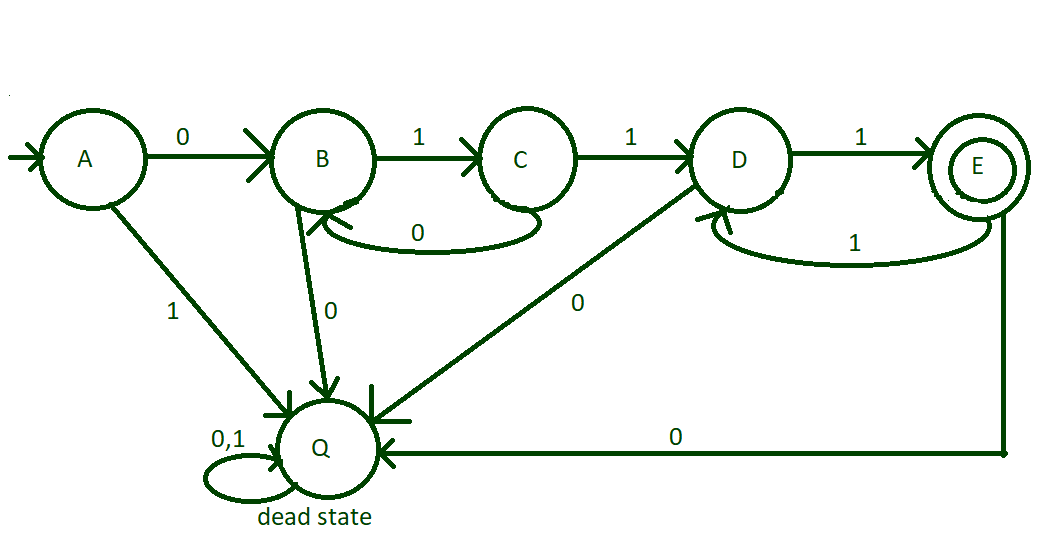# Build a DFA to accept a binary string containing “01” i times and “1” 2j times

• Last Updated : 07 Jun, 2021

Given a binary string str, the task is to build a DFA that accepts given binary string if it contains “01” i times and “1” 2j times, i.e.,Examples:

Input: str = “011111”
Output: Accepted
Explanation:
The string follows the language as: (01)1(1)2*2

Input: str = “01111”
Output: Not Accepted

DFA or Deterministic Finite Automata is a finite state machine which accepts a string(under some specific condition) if it reaches a final state, otherwise rejects it.
In DFA, there is no concept of memory, therefore we have to check the string character by character, beginning with the 0th character. The input set of characters for the problem is {0, 1}. For a DFA to be valid, there must a transition rule defined for each symbol of the input set at every state to a valid state. Therefore, the following steps are followed to design the DFA:

1. Create initial stage and make the transition of 0 and 1 to next possible state.
2. Transition of 0 is always followed by transition of 1.
3. Make an initial state and transit its input alphabets, i.e, 0 and 1 to two different states.
4. Check for acceptance of string after each transition to ignore errors.
5. First, make DfA for minimum length string then go ahead step by step.
6. Define Final State(s) according to the acceptance of string.

Step by Step Approach to design a DFA:

• Step 1: Minimum possible acceptable string is 0111, i.e, (01)1 (11)1. So, create an initial state “A” that make transition of 0 to state “B” and then transition of 1 from “B” to state “C” then transition of 1 from “C” to “D”, then transition of 1 from “D” to “E” as shown in diagram make this stage “E” is final state.• Step 2: Now, think about the string having consecutive (01) and then followed by consecutive (11) to end the string. Hence, when i>1, make a transition of “0” from state “C” to state “B” and make a transition of “1” from the state “E” to state “D”. Hence, strings like 010111, 011111, 0101111111, etc. are acceptable now.• Step 3: We have done with all kind of strings possible to accept. But, there are few input alphabets which are not transited to any of the states. In this case, all these kind of input will be sent to some dead state to block their further transitions that are not acceptable. Input alphabets of the dead state will be sent to the dead state itself. Therefore, the final design of the DFA is:Below is the implementation of the above approach:

## Java

 // Java code for the above DFAimport java.util.*; class GFG{   // Function for the state Astatic void checkstatea(String n){  if (n.length() % 2 != 0 ||      n.length() < 4)    System.out.print("string not accepted");  else  {       int i = 0;         // State transition to B    // if the character is 0    if (n.charAt(i) == '0')      stateb(n.substring(1));    else      System.out.print("string not accepted");  }}  // Function for the state Bstatic void stateb(String n){  int i = 0;     if (n.charAt(i) == '0')    System.out.print("string not accepted");    // State transition to C  // if the character is 1  else    statec(n.substring(1));}   // Function for the state Cstatic void statec(String n){  int i = 0;     // State transition to D  // if the character is 1  if (n.charAt(i) == '1')    stated(n.substring(1));    // State transition to B  // if the character is 0  else    stateb(n.substring(1));}  // Function for the state Dstatic void stated(String n){  int i = 0;     if (n.length() == 1)  {    if (n.charAt(i) == '1')      System.out.print("string accepted");    else      System.out.print("string not accepted");  }  else  {         // State transition to E    // if the character is 1    if (n.charAt(i) == '1')      statee(n.substring(1));    else      System.out.print("string not accepted");  }}  // Function for the state E    static void statee(String n){  int i = 0;     if (n.length() == 1)  {    if (n.charAt(i) == '0')      System.out.print("string not accepted");    else      System.out.print("string accepted");  }  else  {    if (n.charAt(i) == '0')      System.out.print("string not accepted");         stated(n.substring(1));  }}      // Driver codepublic static void main(String []args){     // Take string input  String n ="011111";    // Call stateA to check the input  checkstatea(n);}} // This code is contributed by pratham76

## Python3

 # Python3 program for the given# language # Function for the state Adef checkstatea(n):    if(len(n)%2!=0 or len(n)<4):        print("string not accepted")    else:           i=0         # State transition to B        # if the character is 0        if(n[i]=='0'):            stateb(n[1:])        else:            print("string not accepted") # Function for the state Bdef stateb(n):    i=0    if(n[i]=='0'):        print("string not accepted")     # State transition to C    # if the character is 1    else:        statec(n[1:]) # Function for the state Cdef statec(n):    i=0     # State transition to D    # if the character is 1    if(n[i]=='1'):        stated(n[1:])     # State transition to B    # if the character is 0    else:        stateb(n[1:]) # Function for the state Ddef stated(n):    i=0    if(len(n)==1):        if(n[i]=='1'):            print("string accepted")        else:            print("string not accepted")    else:         # State transition to E        # if the character is 1        if(n[i]=='1'):            statee(n[1:])        else:            print("string not accepted")   # Function for the state E    def statee(n):    i=0    if(len(n)==1):        if(n[i]=='0'):            print("string not accepted")        else:            print("string accepted")              else:        if(n[i]=='0'):            print("string not accepted")        stated(n[1:])            # Driver codeif __name__ == "__main__":     n = "011111"    checkstatea(n)

## C#

 // C# code for the above DFAusing System;using System.Collections;using System.Collections.Generic;class GFG{     // Function for the state Astatic void checkstatea(string n){  if(n.Length % 2 != 0 ||     n.Length < 4)    Console.Write("string not accepted");  else  {       int i = 0;     // State transition to B    // if the character is 0    if(n[i] == '0')      stateb(n.Substring(1));    else      Console.Write("string not accepted");  }} // Function for the state Bstatic void stateb(string n){  int i = 0;  if(n[i] == '0')    Console.Write("string not accepted");   // State transition to C  // if the character is 1  else    statec(n.Substring(1));}  // Function for the state Cstatic void statec(string n){  int i = 0;   // State transition to D  // if the character is 1  if(n[i] == '1')    stated(n.Substring(1));   // State transition to B  // if the character is 0  else    stateb(n.Substring(1));} // Function for the state Dstatic void stated(string n){  int i = 0;  if(n.Length == 1)  {    if(n[i] == '1')      Console.Write("string accepted");    else      Console.Write("string not accepted");  }  else  {    // State transition to E    // if the character is 1    if(n[i] == '1')      statee(n.Substring(1));    else      Console.Write("string not accepted");  }} // Function for the state E    static void statee(string n){  int i = 0;  if(n.Length == 1)  {    if(n[i] == '0')      Console.Write("string not accepted");    else      Console.Write("string accepted");  }  else  {    if(n[i] == '0')      Console.Write("string not accepted");    stated(n.Substring(1));  }}     // Driver codepublic static void Main(string []args){  // Take string input  string n ="011111";   // Call stateA to check the input  checkstatea(n);}} // This code is contributed by rutvik_56

## Javascript

 

Output:

string accepted

My Personal Notes arrow_drop_up